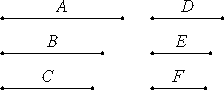# Proposition 14

If there are any number of numbers, and others equal to them in multitude, which taken two and two together are in the same ratio, then they are also in the same ratio ex aequali.

Let there be as many numbers as we please A, B, and C, and others equal to them in multitude D, E, and F, which taken two and two are in the same ratio, so that A is to B as D is to E, and B is to C as E is to F.

I say that, ex aequali A is to C as D is to F.VII.13

Since A is to B as D is to E, therefore, alternately A is to D as B is to E.

Again, since B is to C as E is to F, therefore, alternately B is to E as C is to F. But B is to E as A is to D, therefore A is to D as C is to F. Therefore, alternately A is to C as D is to F.

Therefore, if there are any number of numbers, and others equal to them in multitude, which taken two and two together are in the same ratio, then they are also in the same ratio ex aequali.

Q.E.D.

## Guide

This is the numerical analogue of V.22 for magnitudes. It says that

 if a1 : a2 = b1 : b2 a2 : a3 = b2 : b3 ... and an-1 : an = bn-1 : bn then a1 : an = b1 : bn

Euclid takes n to be 3 in his proof.

The proof is straightforward, and a simpler proof than the one given in V.22 for magnitudes.

Note that at one point, the missing analogue of proposition V.11 is used: from the two proportions B : E = C : F and B : E = A : D, the conclusion A : D = C : F is drawn. Similar missing analogues of propositions from Book V are used in other proofs in book VII. See, for instance, VII.19 where V.7 and V.9 are used. That could mean that when Eudoxus developed the material for Book V he was more careful than his predecessors who created Book VII.

This proposition allows the use of extended proportions such as

a : b : c = d : e : f

as an abbreviation for three ordinary proportions.

This proposition is used occasionally in Books VIII and IX starting with VIII.1.